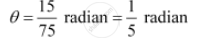CBSE (Arts) Class 11CBSE
Share

# Find the Angle in Radian Though Which a Pendulum Swings If Its Length is 75 Cm and the Tip Describes an Arc of Length 15 Cm - CBSE (Arts) Class 11 - Mathematics

#### Question

Find the angle in radian though which a pendulum swings if its length is 75 cm and the tip describes an arc of length

15 cm

#### Solution

We know that in a circle of radius r unit, if an arc of length l unit subtends an angle θ radian at the centre, then  theta = l/r

It is given that r = 75 cm

Here, = 15 cmIs there an error in this question or solution?

#### APPEARS IN

NCERT Solution for Mathematics Textbook for Class 11 (2018 to Current)
Chapter 3: Trigonometric Functions
Q: 7.2 | Page no. 55

#### Video TutorialsVIEW ALL 

Solution Find the Angle in Radian Though Which a Pendulum Swings If Its Length is 75 Cm and the Tip Describes an Arc of Length 15 Cm Concept: Concept of Angle.
S